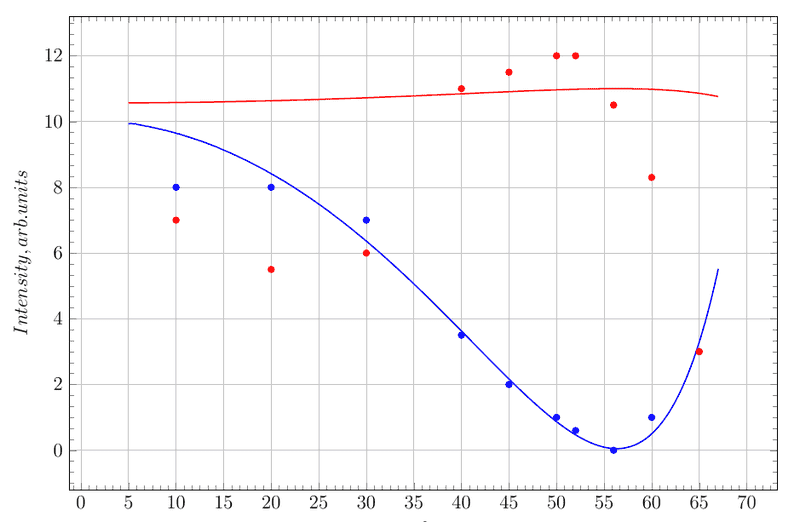# Intensity of p-polarized light through stack of plates

• sergiokapone

#### sergiokapone

As one know, the intensity Fresnel equations
for the reflected p-polarized light
\begin{equation}\label{a}
\frac{I_{p_{refl}}}{I_{0p}}=\frac {\tan^{2}(i-r)}{{\tan^{2}(i+r)}}
\end{equation}

and for the refracted one is

\begin{equation}\label{b}
\frac{I_{p_{refr}}}{I_{0p}}=1 - \frac {\tan^{2}(i-r)}{{\tan^{2}(i+r)}}
\end{equation}

where $i$ - angle of incidence, $r$ - angle of refraction, $I_{op}$ -intensity of incident p-polarized light.

Suppose, we sent p-polarized light to a stack of plates (10 plates with $n = 1.5$).
I expected the intensity of reflected p-polarized light subject to equation (1) and also the intensity of p-polarized light passed across stack of plates subject to equation (2) with some downgrading due to absorption.

I get an experimental data in picture:%====================================
\begin{filecontents}{plate.dat}
angle refracted reflected
7 8 10
5.5 8 20
6 7 30
11 3.5 40
11.5 2 45
12 1 50
12 0.6 52
10.5 0 56
8.3 1 60
3 3 65
\end{filecontents}

The best fit reflected p-polarized light (blue line) is

\begin{equation}\label{fita}
\frac{I_{p_{refl}}}{250}=\frac {\tan^{2}(i-r)}{{\tan^{2}(i+r)}}
\end{equation}

It seems reasonable.
But fit with equatuion (2) looks bad (red line) :
\begin{equation}\label{fitb}
\frac{I_{p_{refr}}}{1/n\cdot 250}=1 - \frac {\tan^{2}(i-r)}{{\tan^{2}(i+r)}}
\end{equation}

I have no idea how to explain it.